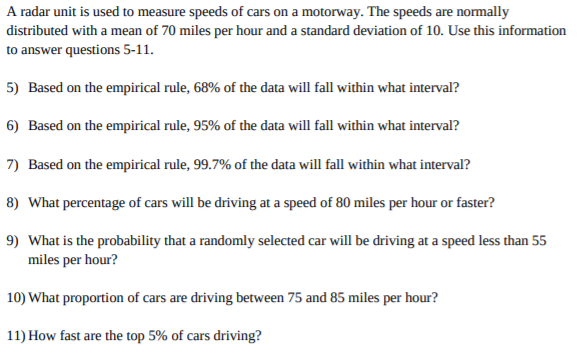# A radar unit is used to measure speeds of cars on a motorway. The speeds are normallydistributed with a mean of 70 miles per hour and a standard deviation of 10. Use this informationto answer questions 5-11.5) Based on the empirical rule, 68% of the data will fall within what interval?6) Based on the empirical rule, 95% of the data will fall within what interval?7) Based on the empirical rule, 99.7% of the data will fall within what interval?8) What percentage of cars will be driving at a speed of 80 miles per hour or faster?9) What is the probability that a randomly selected car will be driving at a speed less than 55miles per hour?10) What proportion of cars are driving between 75 and 85 miles per hour?11) How fast are the top 5% of cars driving?

Question
39 viewshelp_outlineImage TranscriptioncloseA radar unit is used to measure speeds of cars on a motorway. The speeds are normally distributed with a mean of 70 miles per hour and a standard deviation of 10. Use this information to answer questions 5-11. 5) Based on the empirical rule, 68% of the data will fall within what interval? 6) Based on the empirical rule, 95% of the data will fall within what interval? 7) Based on the empirical rule, 99.7% of the data will fall within what interval? 8) What percentage of cars will be driving at a speed of 80 miles per hour or faster? 9) What is the probability that a randomly selected car will be driving at a speed less than 55 miles per hour? 10) What proportion of cars are driving between 75 and 85 miles per hour? 11) How fast are the top 5% of cars driving? fullscreen
check_circle

Step 1

Hey there! Thank you for posting the question. Since there are multiple questions posted, we will answer first question. If you want any specific question to be answered then please submit that ques...

### Want to see the full answer?

See Solution

#### Want to see this answer and more?

Solutions are written by subject experts who are available 24/7. Questions are typically answered within 1 hour.*

See Solution
*Response times may vary by subject and question.
Tagged in

### Statistics